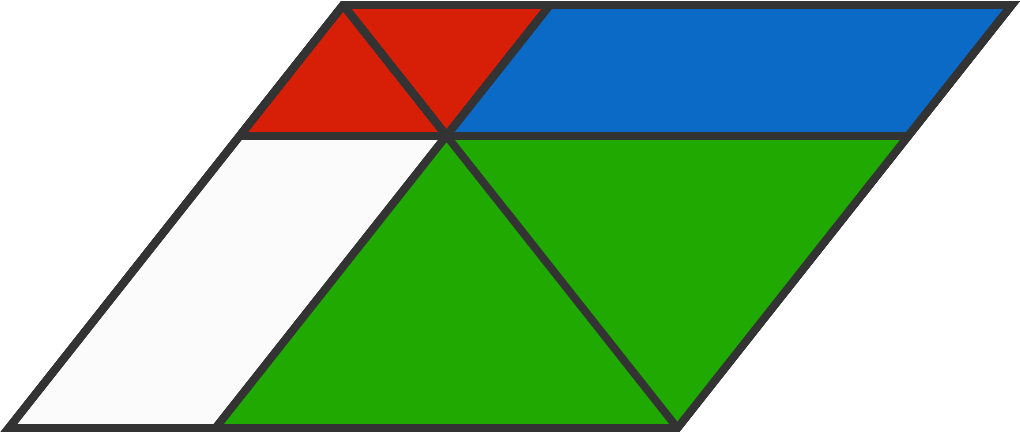# Parellelogram Geometric Progression

Two segments parallel to the sides of a parallelogram are drawn through a point on the parallelogram's diagonal.

The area of the entire figure is 2009, and the areas of the red, blue, and green sections are distinct integers which form a geometric progression in that order.

What is the maximum possible area of the red section?Note: The diagram is not drawn to scale.

×